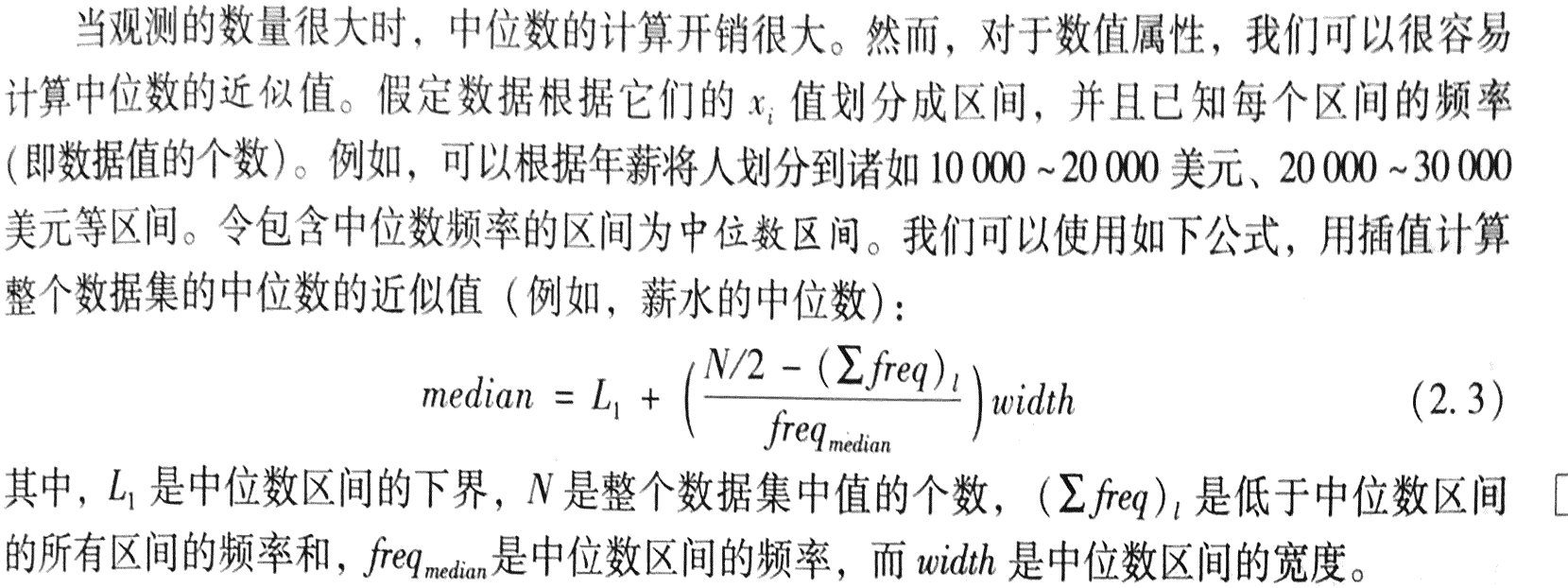• ## 中位数计算

千次阅读 2016-09-29 18:24:07
题目中位数计算。选择你熟悉的编程语言实现教材P31上公式（2.3）的算法，用于估算大量数据的中位数。下载测试数据文件（1百万条数据，中位数为49899），选择不同的区间大小（width），考察计算结果与真实中位数的...
题目

中位数计算。选择你熟悉的编程语言实现教材P31上公式（2.3）的算法，用于估算大量数据的中位数。下载测试数据文件（1百万条数据，中位数为49899），选择不同的区间大小（width），考察计算结果与真实中位数的误差。

公式代码实现

matlab

clear all;
clc;

N = 1000000;
width = 100;    %区间长度
%此处可直接使用median(a)求出中位数49899

max = max(a);       %用其他语言的话,最值可用冒泡排序求出
min = min(a);
num = (max - min) / width;
num = round(num);       %向上取整
count = zeros(num,1);   %保存各区间的个数

%计数
for i = 1:N
count(floor((a(i)-min)/width+1)) = count(floor((a(i)-min)/width+1)) + 1;
end

%确定中位数所在的区间位置index
%计算该区间以前的频数和sigma
sigma = 0;
index = 1;
while N/2-sigma>0
sigma = sigma + count(index);
index = index +1;
end
index = index -1;
sigma = sigma - count(index);

%根据公式计算中位数
L1 = min + width*(index-1);
median = L1 +((N/2-sigma)/count(index)) * width;
median = fix(median);


C

#include<stdio.h>
const int width=100;    //区间长度
void main(){
FILE *fp1,*fp2;
int a,max=2000,min=2000,N=0,median;
if( (fp1 = fopen("rand1m.txt","r"))==NULL )
printf("error");

//取出最大值与最小值
while(fscanf(fp1,"%d%*[^0-9]",&a)>0){
if(a>=max)
max = a;
if(a<=min)
min = a;
N++;
}
fclose(fp1);

int num = (max - min) / width +1;   //区间个数
int* count = new int[num];      //区间频数
for(int i=0; i<num; ++i)
count[i]=0;

if( (fp2 = fopen("rand1m.txt","r"))==NULL )
printf("error");

//计数
while(fscanf(fp2,"%d%*[^0-9]",&a)>0){
count[(a-min)/width]++;
}
fclose(fp2);

//确定区间index 统计index前所有区间频数和sigma
int sigma = 0;
int index = 0;
while(N/2 - sigma >0){
sigma += count[index];
index ++;
}
index --;
sigma -= count[index];

//套用公式
int L1 = min + width*index;     //区间下限
double rate = (1.0) * (N/2-sigma) / count[index];
median = L1 + rate * width;

printf("L1=%d\nmedian=%d\n",L1,median);

}

展开全文• 1、一个子集中的中位数 COUNT(x.cashmoneys)为奇数 SELECT x.cashmoneys FROM (select distinct cashmoneys from apprexpense) x, (select distinct cashmoneys f
1、一个子集中的中位数
COUNT(x.cashmoneys)为奇数
SELECT x.cashmoneys FROM (select distinct cashmoneys from apprexpense) x, (select distinct cashmoneys from apprexpense) y
GROUP BY x.cashmoneys
HAVING SUM(sign(1-sign(x.cashmoneys-y.cashmoneys)))=(COUNT(x.cashmoneys)+1)/2
COUNT(x.cashmoneys)为偶数
select avg(z.cashmoneys) from
(
SELECT x.cashmoneys FROM (select distinct cashmoneys from apprexpense) x, (select distinct cashmoneys from apprexpense) y
GROUP BY x.cashmoneys
HAVING SUM(sign(1-sign(x.cashmoneys-y.cashmoneys)))=COUNT(x.cashmoneys)/2
or SUM(sign(1-sign(x.cashmoneys-y.cashmoneys)))=(COUNT(x.cashmoneys)/2+1)
) z
2、多个子集中的中位数
COUNT(x.cashmoneys)为奇数
SELECT x.type,x.cashmoneys FROM (select distinct type,cashmoneys from apprexpense) x inner join (select distinct type,cashmoneys from apprexpense) y on x.type=y.type
GROUP BY x.type,x.cashmoneys
HAVING SUM(sign(1-sign(x.cashmoneys-y.cashmoneys)))=(COUNT(x.cashmoneys)+1)/2

展开全文sqlserver join
• ## Python计算中位数 numpy.median

万次阅读 多人点赞 2017-07-04 17:46:48
计算沿指定轴的中位数 返回数组元素的中位数其函数接口为：median(a, axis=None, out=None, overwrite_input=False, keepdims=False)其中各参数为： a：输入的数组； axis：计算哪个轴上的中位数，比如...
numpy模块下的median作用为：
计算沿指定轴的中位数
返回数组元素的中位数
其函数接口为：
median(a,
axis=None,
out=None,
overwrite_input=False,
keepdims=False)

其中各参数为：
a：输入的数组；
axis：计算哪个轴上的中位数，比如输入是二维数组，那么axis=0对应行，axis=1对应列，如果对于二维数组不指定长度，将拉伸为一唯计算中位数；
out：用于放置求取中位数后的数组。 它必须具有与预期输出相同的形状和缓冲区长度；
overwrite_input :一个bool型的参数，默认为Flase。如果为True那么将直接在数组内存中计算，这意味着计算之后原数组没办法保存，但是好处在于节省内存资源，Flase则相反；
keepdims：一个bool型的参数，默认为Flase。如果为True那么求取中位数的那个轴将保留在结果中；
>>> a = np.array([[10, 7, 4], [3, 2, 1]])
>>> a
array([[10,  7,  4],
[ 3,  2,  1]])
>>> np.median(a)
# 1，2，3，4，7，10
# (3+4)/2 = 3.5
3.5
>>> np.median(a, axis=0)
#(10+3)/2 = 6.5
#(7+2)/2 = 4.5
#(4+1)/2 = 2.5
array([ 6.5,  4.5,  2.5])
>>> np.median(a, axis=1)
#奇数个数中位数
array([ 7.,  2.])
>>> m = np.median(a, axis=0)
>>> out = np.zeros_like(m)
>>> np.median(a, axis=0, out=m)
array([ 6.5,  4.5,  2.5])
>>> m
array([ 6.5,  4.5,  2.5])
>>> b = a.copy()
>>> np.median(b, axis=1, overwrite_input=True)
array([ 7.,  2.])
>>> assert not np.all(a==b)
>>> b = a.copy()
>>> np.median(b, axis=None, overwrite_input=True)
3.5



展开全文python numpy median
• 最近工作需要 要求把python的代码写成java版本，python中有一个np.median（）求中位数的方法，java决定手写一个 先说说什么是中位数中位数就是中间的那个数， 如果一个集合是奇数个，那么中位数就是按大小排列...
最近工作需要 要求把python的代码写成java版本，python中有一个np.median（）求中位数的方法，java决定手写一个

先说说什么是中位数：

中位数就是中间的那个数，

如果一个集合是奇数个，那么中位数就是按大小排列后，最中间那个数，

如果一个集合是偶数个，那么中位数就是按大小排列后，最中间那2个数的平均数。

比如：

1，2，3，4，5  那中位数就是3

1，2，3，4，5，6 那中位数就是 （3+4）/2 = 3.5

知道逻辑后方法就很简单了 下面是代码

public static void main(String[] args) {
List<Integer> total = new ArrayList<Integer>();
double a = median(total);
System.out.println(a);
}
private static double median(List<Integer> total) {
double j = 0;
//集合排序
Collections.sort(total);
int size = total.size();
if(size % 2 == 1){
j = total.get((size-1)/2);
}else {
//加0.0是为了把int转成double类型，否则除以2会算错
j = (total.get(size/2-1) + total.get(size/2) + 0.0)/2;
}
return j;
}

1. 方法内先判断集合是奇数还是偶数，如果是奇数那么就是第n+1/2个数 ，也就是下标为n-1/2的值，

如果是偶数 就是第n/2和n/2+1的数的平均值 也就是下标为n/2-1和n/2的平均值

2. 该方法传入的是list集合  如果为数组  可以先用Arrays.aslist()方法转换后传入
展开全文java
• ## 算法 - 求n个数的中位数（C++）

万次阅读 多人点赞 2019-02-28 10:19:02
分享一个大牛的人工智能教程。零基础！通俗易懂！风趣幽默！希望你也加入到人工智能的队伍中来！... /* * 求n个数的中位数 - C++ - by Chimomo ... * 计算有限个数的数据的中位数的方法是：把所有的同类...算法 C++
• 1)计算平均数/众数/中位数 在调查数据分析（Survey data analysis）中经常需要计算平均数、中位数和众数。用函数编程计算40个输入数据（是取值1—10之间的任意整数）的平均数（Mean）、中位数（Median）和众数（Mode...c语言
• 41.1 数据流中的中位数 题目描述 如何得到一个数据流中的中位数？如果从数据流中读出奇数个数值，那么中位数就是所有数值排序之后位于中间的数值。如果从数据流中读出偶数个数值，那么中位数就是所有数值排序之后...
• 什么是中位数？ 数值型数组的中位数是在数据排序后位于数组中间项的值。如果数组有偶数个元素，中位数就是最中间的两个数值的平均数。 中位数对于了解“我的值是否位于中间？”非常有用。比如，我在学校的最后...奇数 mysql 过程 元素
• 常规（数据量不大的情况）求中位数和基于插值计算中位数的方法 在学数学时我们学到过求中位数的方法，在数据个数为偶数时找最中间的两个数然后求平均数如果数据个数为奇数时则只需找数据个数一半的那位上的数字...大数据 java 算法
• 这个解决方法非常巧妙，它利用了取反数和为1的特性，通过列表负索引来获得列表中位数。 对 return (data[half] + data[~half]) / 2 的解释： 排序后得到序列[1,2,3,4,5,6]，其列表长度为偶数，中位数由列表中间...
• 中位数（Median）又称中值，统计学中的专有名词，是按顺序排列的一组数据中居于中间位置的数，代表一个样本、种群或概率分布中的一个数值，其可将数值集合划分为相等的上下两部分。对于有限的数集，可以通过把所有...hive udaf
• 计算一串数组的均值、中位数、标准差 #!/usr/bin/env python #-*- coding:utf-8 -*- ''' @author : FIGTHING @file : DataMining.py @function: @software: Pycharm @time : 2019/06/13/15:40 ''' import numpy as...
• 希望你也加入到人工智能的队伍来！请点击http://www.captainbed.net /* * Created by Chimomo * * Let X[0...n-1] and Y[0...n-1] be the two arrays, each containing n numbers already in the so...算法 C++
• pgsql实现中位数、众数和平均数的计算。 pgsql中不支持median函数，说以只能用percentile_disc实现 如下 select -- 众数 mode() within group ( order by active_days ) as mode_active_days -- 中位数 ,...postgresql sql 数据库
• 中位数定义为，将一个数列排序后位于中间的数值（数列长度为奇数时，取正中间的数，长度为偶数时，去中间的两个数的平均） 众数定义为，在一个数列中，出现次数最多的那个数值。 python代码实现：#计算平均数 def ...python 数学
• #计算中位数 def count_median(lis): if len(lis) % 2 == 0: mid = float((lis[len(lis) / 2] + lis[len(lis) / 2 - 1])) / 2 else: mid = lis[len(lis) / 2] return mid #计算上下四分位数 def count_...
• ## c++：计算中位数

千次阅读 2018-04-27 08:50:17
输入数据 第一行为一个整数 t (1≤t≤100)t (1≤t≤100)，表示数据的组。接下来对于每组数据： 第一行为一个整数 n (1≤n≤500)n (1≤n≤500)，表示参加考试的人数。 第二行有 nn 个整数 a1,a2,…an (0≤ai≤...
• 对于元素个数不同的列表而言，中位数计算方式分为如下两种。 （1）若列表中元素的个数为奇数，则中位数为排序后列表中间位置的那个数。 （2）若列表中元素的个数为偶数，则中位数为排序后列表中间位置的两个数的...
• ## SQL计算中位数

万次阅读 2012-10-23 11:03:53
可以查找到中间的项，count(*)+1，这个1必须要添加，总项假如为奇数7，则count(*)+1 / 2 = 4，假如是6则为3，都是满足的，如果不+1的话，对奇数项则不满足，如7，count(*) /2 = 3，而我们要查找的则是位于4的位置 ...sql div
• 中位数即2分位数，那么同样可以使用该函数计算。具体使用方如下： percentile：percentile(col, p) col是要计算的列（值必须为int类型），p的取值为0-1，若为0.5，那么就是2分位数，即中位数。 percentile_approx...hadoop cloudera hive
• NumPy中可以通过median函数计算中位数；通过var函数计算方差。方差是指各个数据与所有数据算术平均数的离差平方和除以数据个数所得到的值。  计算中位数和方差实例： #NumPy常用函数：计算中位数和方差 from ...
• 众数、中位数、分位数 使用 numpy 的 median 函数可以得到其中位数，quantile 函数可以得到其分位数，但 numpy 包目前还没有计算众数的函数。例如： >>> a = [8, 19, 34, 9, 18] >>> np.median(a) # 得到数组 a 的...python 峰度
• ## 中位数

千次阅读 2013-03-19 10:23:57
中位数（Medians）统计学名词，是指将统计总体当中的各个变量值按大小顺序排列起来，形成一个数列，处于变量数列中间位置的变量值就称为中位数，用Me表示。当变量值的项数N为奇数时，处于中间位置的变量值即为中位数...
• ## 四分位数计算方法

千次阅读 2019-07-20 12:45:00
下四分位数的位置Q1=（n+1）*0.25 中位数的位置Q2=（n+1）*0.5 上四分位数的位置Q3=（n+1）*0.75 转载于:https://www.cnblogs.com/bravesunforever/p/11217344.html...
• ## mysql_如何用mysql计算每组的中位数

万次阅读 多人点赞 2018-01-19 21:19:10
有一天，我不得不计算MySQL中每个组的中位数。 事实证明这并不像听起来那么简单，主要是因为在MySQL中不存在median（）函数。 经过一些反复试验和一些有用的提示，我想到了。 尤其对于那些不熟悉MySQL的人来说，这里...
• ## 中位数（C语言）

万次阅读 2017-06-19 17:50:46
计算有限个数的数据的中位数的方法是：把所有的同类数据按照大小的顺序排列。如果数据的个数是奇数，则中间那个数据就是这群数据的中位数；如果数据的个数是偶数，则中间那2个数据的算术平均值就是这群数据的中位数...c语言
• ## python求均值、中位数、众数的方法

万次阅读 多人点赞 2018-05-26 16:18:58
首先需要数据源，这里随便写了一个：nums = [1,2,3,4]求均值和中位数均可以使用numpy库的方法： #均值 np.mean(nums) #中位数 np.median(nums)求众数方法一：在numpy中没有直接的方法，但是也可以这样实现：import ...python numpy...